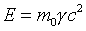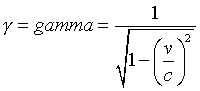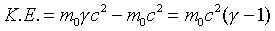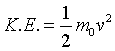QUESTION #121

# Does Einstein's mass-energy equation (E = mc2) imply that the mass of an object increases when we give it enough energy, be it kinetic or otherwise?

No. First, we should define the mass. Mass is defined with respect to a frame where the velocity of this object is zero, and often called as 'rest mass'. In this equation 'm' is not the rest mass. If we denote rest mass with m0, then the equation should be written as:Where gamma is equal to:And, this equation gives the energy of the particle with mass m, with respect to a frame whose travelling with speed

.

So, the kinetic energy can be calculated, by finding the energy difference between each case; when the particle is at rest w.r.t. frame that gamma = 1, and when it travels with some speed.Note that gamma is always greater then 1. And, when v is much smaller than c, the K.E. equation can be approximated with a great precision by the non-relativistic K.E. equation:As a result, 'm' in this equation is actually 'm0 * gamma', where m0 is the mass of the object, gamma is a unitless number which depends on the velocity of the object, w.r.t. the frame where the measurements are done.
Answered by: Taylan Akdogan, Physics, Ph.D. candidate, M.I.T.

I respectfully, disagree with the answer to the above question.

Mass m in the equation E = mc2 is equal to the rest mass (m0) times the factor gamma. Gamma has the value 1 when v = 0, and increases toward infinity as v approaches c. That is, the mass in E = mc2, which is clearly the mass the questioner is concerned about, does indeed increase as an object acquires energy due to motion = kinetic energy. E = mc2 clearly implies that, as total relativistic energy E increases, the total (rest and relativistic kinetic) mass increases correspondingly!

It is this _total_ mass that has to be reckoned with when interacting with the object. For example, the mass of the electrons in the electron beam of a CRT increases due to the acceleration of the electrons toward the face of the tube. This factor has to be taken into account in designing the deflection system that scans the beam across the tube face. Likewise, the considerable increase of the mass of particles in particle accelerators has to be taken into account in the design of accelerators.

Furthermore the answer to the question is not complete. There was also a 'be it kinetic, or otherwise?' The questioner should be informed that, yes indeed, it doesn't make any difference what form of energy is acquired by the object. Kinetic energy is only one possibility. Thermal energy and potential energy (of various kinds) also matter. For example, the binding potential energy between the constituents of the nucleus contributes significantly to the mass of the nucleus. It is precisely the liberation of this mass-due-to-binding-energy that is the basis of nuclear fission reactors and the atomic bomb!
Answered by: Warren F. Davis, Ph.D., President, Davis Associates, Inc., Newton, MA USA